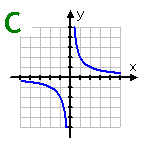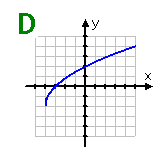Symmetry
Examples ContinuedGraph C: This graph is symmetric about the lines: y = x and y = -x. It is also symmetric about the origin. Because no vertical line can cross the graph more than once, the graph is an odd function.Graph D: This graph of a square-root function shows no symmetry. Although it is a function, it would not be considered even or odd.

Listen